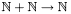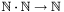#Boost C++ Libraries

...one of the most highly regarded and expertly designed C++ library projects in the world.

## Quantities

Heterogeneous Operators
Conversions
Construction and Conversion of Quantities

A quantity is defined as a value of an arbitrary value type that is associated with a specific unit. For example, while meter is a unit, 3.0 meters is a quantity. Quantities obey two separate algebras: the native algebra for their value type, and the dimensional analysis algebra for the associated unit. In addition, algebraic operations are defined between units and quantities to simplify the definition of quantities; it is effectively equivalent to algebra with a unit-valued quantity.

Quantities are implemented by the `quantity` template class defined in `boost/units/quantity.hpp` :

```template<class Unit,class Y = double> class quantity;
```

This class is templated on both unit type (`Unit`) and value type (`Y`), with the latter defaulting to double-precision floating point if not otherwise specified. The value type must have a normal copy constructor and copy assignment operator. Operators +, -, *, and / are provided for algebraic operations between scalars and units, scalars and quantities, units and quantities, and between quantities. In addition, integral and rational powers and roots can be computed using the `pow`<R> and `root`<R> functions. Finally, the standard set of boolean comparison operators ( ```==, !=, <, <=, >, and >=``` ) are provided to allow comparison of quantities from the same unit system. All operators simply delegate to the corresponding operator of the value type if the units permit.

### Heterogeneous Operators

For most common value types, the result type of arithmetic operators is the same as the value type itself. For example, the sum of two double precision floating point numbers is another double precision floating point number. However, there are instances where this is not the case. A simple example is given by the natural numbers where the operator arithmetic obeys the following rules (using the standard notation for number systems):

••••This library is designed to support arbitrary value type algebra for addition, subtraction, multiplication, division, and rational powers and roots. It uses Boost.Typeof to deduce the result of these operators. For compilers that support `typeof`, the appropriate value type will be automatically deduced. For compilers that do not provide language support for `typeof` it is necessary to register all the types used. For the case of natural numbers, this would amount to something like the following:

```BOOST_TYPEOF_REGISTER_TYPE(natural);
BOOST_TYPEOF_REGISTER_TYPE(integer);
BOOST_TYPEOF_REGISTER_TYPE(rational);
```

### Conversions

Conversion is only meaningful for quantities as it implies the presence of at least a multiplicative scale factor and, possibly, and affine linear offset. Macros for simplifying the definition of conversions between units can be found in `boost/units/conversion.hpp` and `boost/units/absolute.hpp` (for affine conversions with offsets).

The macro `BOOST_UNITS_DEFINE_CONVERSION_FACTOR` specifies a scale factor for conversion from the first unit type to the second. The first argument must be a `base_unit`. The second argument can be either a `base_unit` or a `unit`.

Let's declare a simple base unit:

```struct foot_base_unit : base_unit<foot_base_unit, length_dimension, 10> { };
```

Now, we want to be able to convert feet to meters and vice versa. The foot is defined as exactly 0.3048 meters, so we can write the following

```BOOST_UNITS_DEFINE_CONVERSION_FACTOR(foot_base_unit, meter_base_unit, double, 0.3048);
```

Alternately, we could use the SI length `typedef`:

```BOOST_UNITS_DEFINE_CONVERSION_FACTOR(foot_base_unit, SI::length, double, 0.3048);
```

Since the SI unit of length is the meter, these two definitions are equivalent. If these conversions have been defined, then converting between scaled forms of these units will also automatically work.

The macro `BOOST_UNITS_DEFAULT_CONVERSION` specifies a conversion that will be applied to a base unit when no direct conversion is possible. This can be used to make arbitrary conversions work with a single specialization:

```struct my_unit_tag : boost::units::base_unit<my_unit_tag, boost::units::force_type, 1> {};
// define the conversion factor
BOOST_UNITS_DEFINE_CONVERSION_FACTOR(my_unit_tag, SI::force, double, 3.14159265358979323846);
// make conversion to SI the default.
BOOST_UNITS_DEFAULT_CONVERSION(my_unit_tag, SI::force);
```

### Construction and Conversion of Quantities

This library is designed to emphasize safety above convenience when performing operations with dimensioned quantities. Specifically, construction of quantities is required to fully specify both value and unit. Direct construction from a scalar value is prohibited (though the static member function `from_value` is provided to enable this functionality where it is necessary. In addition, a `quantity_cast` to a reference allows direct access to the underlying value of a `quantity` variable. An explicit constructor is provided to enable conversion between dimensionally compatible quantities in different unit systems. Implicit conversions between unit systems are allowed only when the reduced units are identical, allowing, for example, trivial conversions between equivalent units in different systems (such as SI seconds and CGS seconds) while simultaneously enabling unintentional unit system mismatches to be caught at compile time and preventing potential loss of precision and performance overhead from unintended conversions. Assignment follows the same rules. An exception is made for quantities for which the unit reduces to dimensionless; in this case, implicit conversion to the underlying value type is allowed via class template specialization. Quantities of different value types are implicitly convertible only if the value types are themselves implicitly convertible. The `quantity` class also defines a `value()` member for directly accessing the underlying value.

To summarize, conversions are allowed under the following conditions :

• implicit conversion of `quantity<Unit,Y>` to `quantity<Unit,Z>` is allowed if `Y` and `Z` are implicitly convertible.
• assignment between `quantity<Unit,Y>` and `quantity<Unit,Z>` is allowed if `Y` and `Z` are implicitly convertible.
• explicit conversion between `quantity<Unit1,Y>` and `quantity<Unit2,Z>` is allowed if `Unit1` and `Unit2` have the same dimensions and if `Y` and `Z` are implicitly convertible.
• implicit conversion between `quantity<Unit1,Y>` and `quantity<Unit2,Z>` is allowed if `Unit1` reduces to exactly the same combination of base units as `Unit2` and if `Y` and `Z` are convertible.
• assignment between `quantity<Unit1,Y>` and `quantity<Unit2,Z>` is allowed under the same conditions as implicit conversion.
• `quantity<Unit,Y>` can be directly constructed from a value of type `Y` using the static member function `from_value`. Doing so, naturally, bypasses any type-checking of the newly assigned value, so this method should be used only when absolutely necessary.

Of course, any time implicit conversion is allowed, an explicit conversion is also legal.

Because dimensionless quantities have no associated units, they behave as normal scalars, and allow implicit conversion to and from the underlying value type or types that are convertible to/from that value type.

 Copyright © 2003-2008 Matthias Christian SchabelCopyright © 2007-2010 Steven Watanabe Distributed under the Boost Software License, Version 1.0. (See accompanying file LICENSE_1_0.txt or copy at http://www.boost.org/LICENSE_1_0.txt)